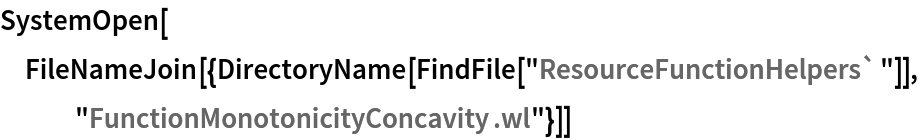Function Repository Resource:

# SignChart

Get a number line diagram with information about where a function is positive, negative, zero or discontinuous

Contributed by: Wolfram|Alpha Math Team
 ResourceFunction["SignChart"][expr, x] creates a number line diagram with information about the sign of the real univariate function expr, written in terms of the variable x.

## Details

ResourceFunction["SignChart"] accepts all of the options of NumberLinePlot, as well as the following:
 FontSize Medium the font size of sign chart labels "PlusColor" the color corresponding to positive points/intervals "MinusColor" the color corresponding to negative points/intervals "ZeroColor" the color corresponding to zero or discontinuous points/intervals Tooltip True whether to include tooltips corresponding to critical values

## Examples

### Basic Examples (1)

Get a sign chart for 2x-10, which is negative for x<5, zero at x=5 and positive for x>5:

 In:=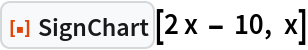Out=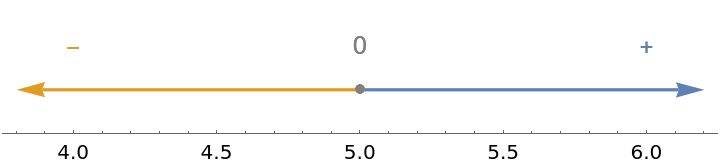### Scope (1)

Discontinuities are indicated on the sign chart with vertical dots:

 In:=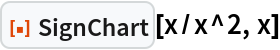Out=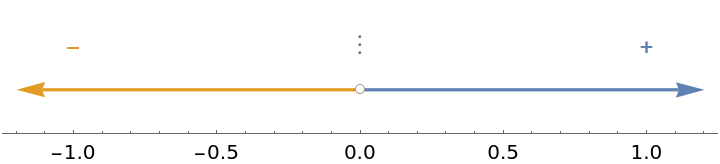### Options (4)

#### FontSize (1)

Use the option FontSize to adjust the size of labels:

 In:=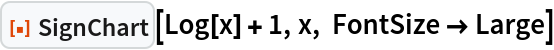Out=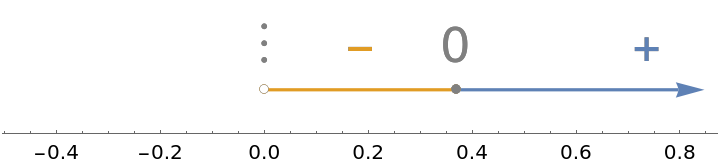#### MinusColor (1)

Use the option "MinusColor" to adjust the color of the negative intervals and minus signs:

 In:=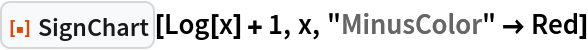Out=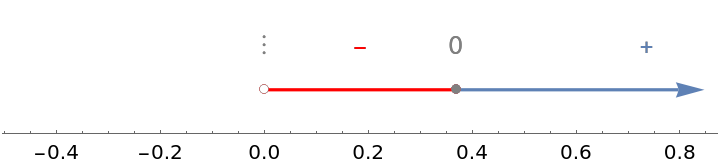#### PlusColor (1)

Use the option "PlusColor" to adjust the color of the positive intervals and plus signs:

 In:=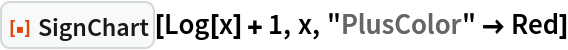Out=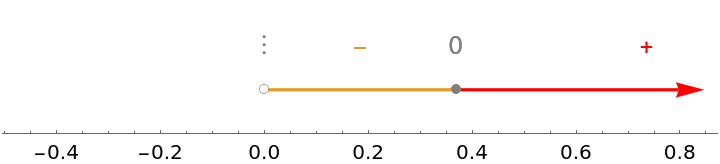#### ZeroColor (1)

Use the option "ZeroColor" to adjust the color of the zero or discontinuous intervals and zeros:

 In:=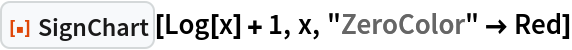Out=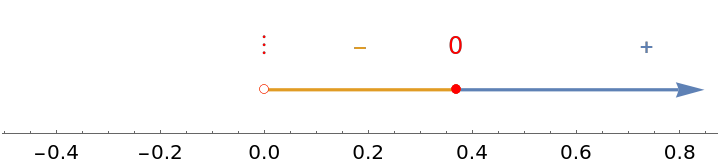### Applications (1)

Sign charts are a useful reasoning tool in many contexts, especially calculus functional analysis. For example, compare the plot of a function to the sign chart of the function's derivative to visualize the first derivative test:

 In:=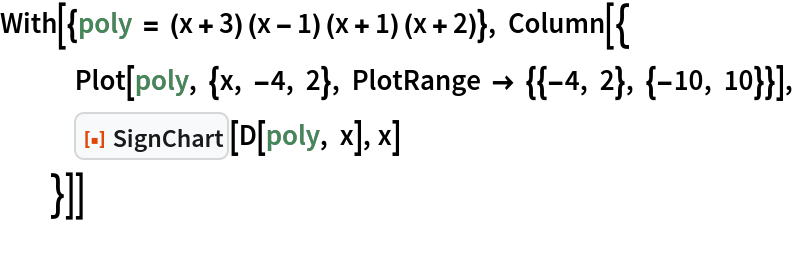Out=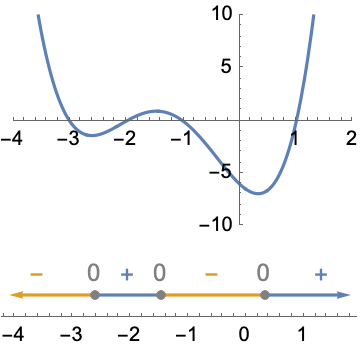### Possible Issues (1)

If there are infinitely many places where a function changes signs, the sign chart displays information for an interval surrounding x=0:

 In:=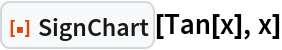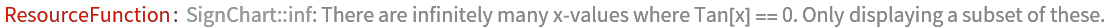Out=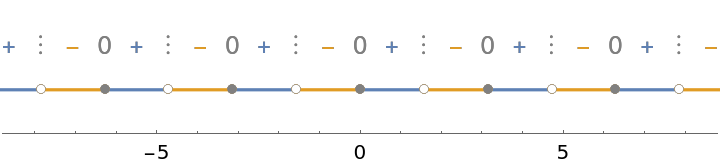## Publisher

Wolfram|Alpha Math Team

## Version History

• 2.0.0 – 23 March 2023
• 1.0.0 – 11 October 2022

## Author Notes

To view the full source code for SignChart, evaluate the following: